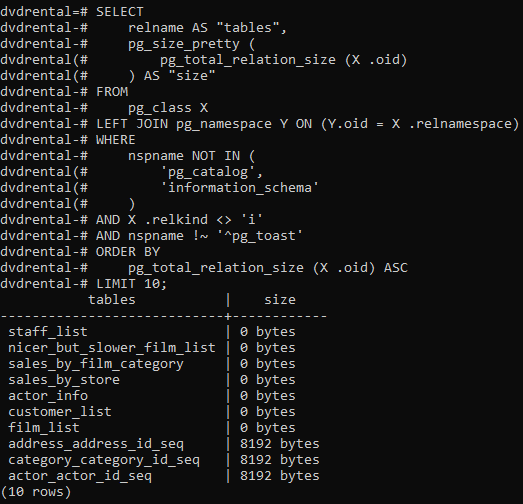Open In App

# PostgreSQL – Size of a Table

In this article, we will look into the function that is used to get the size of the PostgreSQL database table. In this article, we will be using a sample database for reference which is described here and can be downloaded from here.

The pg_relation_size() function is used to get the size of a table.

`Syntax: select pg_relation_size('table_name');`

Example 1:
Here we will query for the size “country” table from the sample dvdrental database using the below command:

`select pg_relation_size('country');`

Output: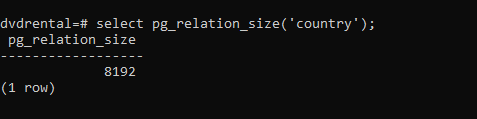To make the result readable, one can use the pg_size_pretty() function. The pg_size_pretty() function takes the result of another function and formats it using bytes, kB, MB, GB or TB as required.

`SELECT pg_size_pretty (pg_relation_size('country'));`

Output: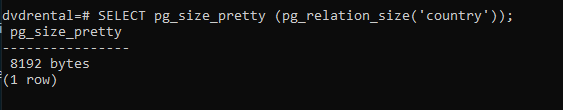Example 2:
Here we will query for the size “customer” table from the sample dvdrental database using the below command:

`SELECT pg_size_pretty (pg_relation_size('customer'));`

Output: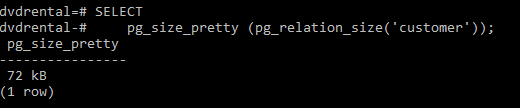Example 3:
Here we will query for the size “film” table from the sample dvdrental database using the below command:

`SELECT pg_size_pretty (pg_relation_size('film'));`

Output: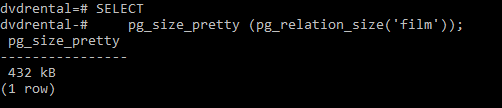Example 4:
Here we will query for the top 10 biggest tables in the dvdrental database.

```SELECT
relname AS "tables",
pg_size_pretty (
pg_total_relation_size (X .oid)
) AS "size"
FROM
pg_class X
LEFT JOIN pg_namespace Y ON (Y.oid = X .relnamespace)
WHERE
nspname NOT IN (
'pg_catalog',
'information_schema'
)
AND X .relkind <> 'i'
AND nspname !~ '^pg_toast'
ORDER BY
pg_total_relation_size (X .oid) ASC
LIMIT 10;```

Output: